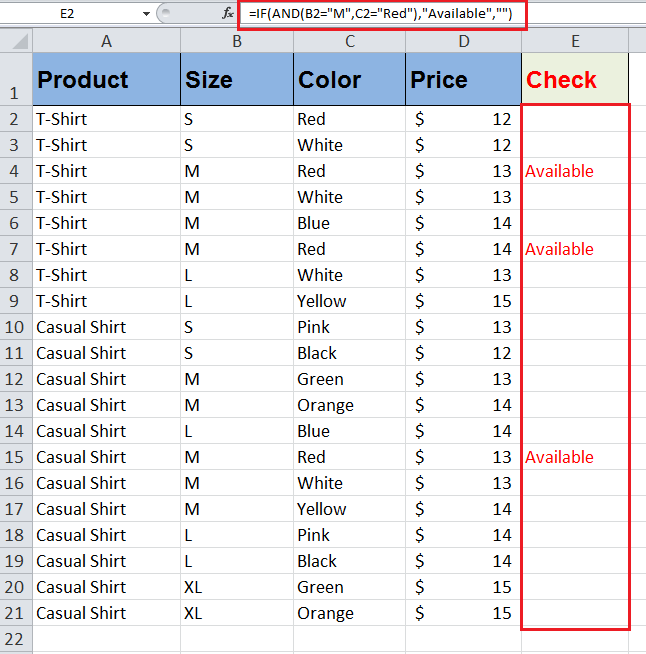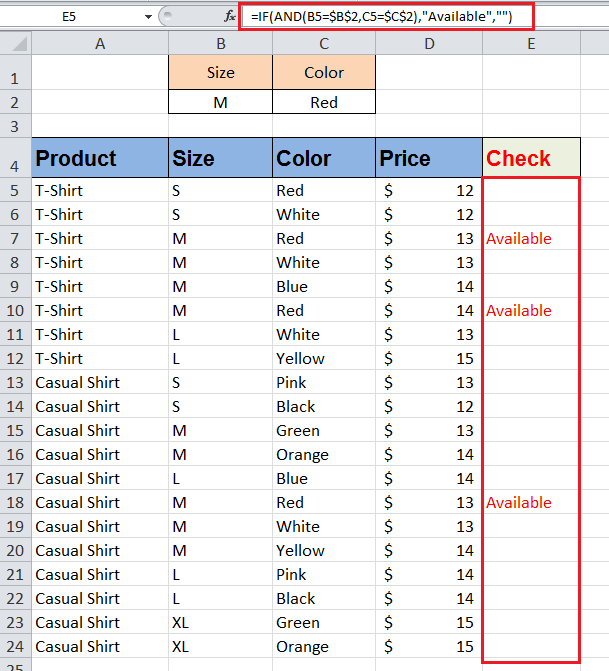Get instant live expert help with Excel or Google Sheets“My Excelchat expert helped me in less than 20 minutes, saving me what would have been 5 hours of work!”

#### Post your problem and you’ll get expert help in seconds.

Your message must be at least 40 characters
Our professional experts are available now. Your privacy is guaranteed.

# Learn How To Use an IF AND Function in Excel

In Excel’s IF statement formula, you face a situation when you need to apply more than one logical test and want to return a value when all the applied logical tests or conditions are TRUE. This is the situation where IF and AND functions come into action as a combined IF AND statement.

## The Excel AND Function Combined with IF

The Excel AND function is one of the logical functions, and it returns TRUE if all conditions are met. Otherwise, it returns FALSE if any of the applied conditions do not meet. The syntax of AND function is;

`AND(logical1, [logical2], …..)`

In the IF statement, the AND function is used in logical_test argument part to test multiple logical conditions, and it returns a value if all conditions are TRUE, else it returns something else. So as a combined IF AND statement the syntax would be;

`IF(AND(logical1, [logical2], ...), [value_if_true], [value_if_false])`

You can see the AND function has replaced logical_test argument of the IF statement because the AND function is itself a logical function. You need to apply all logical tests in the AND function of the IF statement those need to be TRUE to return a value in value_if_true argument, else return another value in value_if_false argument, if any of the applied logical tests are FALSE.

### Example of IF AND Function

Suppose you have a data set of men’s shirts in various sizes and colors. Each product has different prices for each size and color available in stock. You need to check the availability of a certain size and color combination in a long list of product’s sizes and colors combinations.

Using the IF AND statement you need to check the availability of all those products that meet both conditions, like “Available,” otherwise leave the status as a blank value.

You need to supply both logical conditions in the AND function. The AND function will return TRUE if any product meets both conditions, and IF statement will return value “Available” provided in value_if_true argument, otherwise the IF statement will return a blank value (“”), if any of the conditions supplied in the AND function are FALSE, such as;

`=IF(AND(B2="M",C2="Red"),"Available","")`You can also supply logical tests values as hard-coded values as given above or you can use cell references in the AND function containing logical conditions, such as

`=IF(AND(B5=\$B\$2,C5=\$C\$2),"Available","")`

You need to make the cell references absolute that contain logical tests’ values by using \$ sign with column and row references so that these cell references do not change when you copy the formula to other rows containing data as shown above.Still need some help with Excel formatting or have other questions about Excel? Connect with a live Excel expert here for some 1 on 1 help. Your first session is always free.

### Did this post not answer your question? Get a solution from connecting with the expert.Another blog reader asked this question today on Excelchat:
Related blogs
Solution examplesI have a list or people who are ranked in numbers from 3 to 6 I need to recognize the contents of each multiple cells and create an equivalent letter. for that value into another column. e.g. Cell E1 = 3 to show in new cell that row (H1) the letter "C" I have multiple rows with different values in column "E" Can this be done?
Solved by I. J. in 30 minsCould you help me by explaining the IF function?
Solved by G. L. in 24 minsYes hello Im looking for help on conditional formatting I currently have a conditional format for D9>D32 and so on from D9:S20. I need to add an if statement that references cell D4 if the first four characters are 1358 for the rule to apply can you please assist?
Solved by Z. U. in 20 minsA2=50 A3=46 A4=39 =IF(A2>=48,100) =IF(AND(A3<=47,A3>=41),120) =IF(A4<=40,A4*3) Individually this logic formula works with the desired value result. When I nested the IF's the only the first logic statement gave me a value result the other logic statements gave me a "False". Here is the nested formula I created. =IF(A2<=40,A2*3,IF(AND(A2<=47,A2>=41,120),IF(A2>=48,100)))
Solved by C. C. in 11 minsI have a question about Conditional Formatting. =IF('Sheet1'!A1="X",IF('Sheet1'!B1="Y", TRUE, FALSE),FALSE) the set the cell background to red. I would like to make it so that if Sheet1'!B1="Z" I set the back to blue. How can I achieve this.
Solved by B. F. in 39 mins## Subscribe to Excelchat.coAnother blog reader asked this question today on Excelchat: